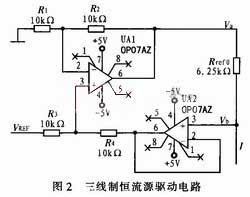﻿ Design of the Drive Circuit of 3 Wire Pt1000_

China sensor manufacturers

# Design of the Drive Circuit of 3 Wire Pt1000

The constant current source driving circuit is responsible for driving the temperature sensor Pt1000 to convert its sensed temperature-varying resistance signal into a measurable voltage signal. In this system, the constant current source required has a constant output current and good temperature stability. The output resistance is very large, the output current is less than 0.5 mA (the upper limit of Pt1000 has no self-heating effect), the load is grounded at one end, and the polarity of the output current can be changed.

Since the influence of temperature on the integrated operational amplifier parameters is not as significant as that of the transistor or FET parameters, the constant current source composed of the integrated operational amplifier has the advantages of better stability and higher constant current performance. Especially in the case where the load end needs to be grounded, it has been widely used. Therefore, the dual op amp constant current source shown in Figure 2 is used. The amplifier UA1 constitutes an adder, UA2 constitutes a follower, and UA1 and UA2 select a low noise, low offset, high open loop gain bipolar operational amplifier OP07.3-wire constant current source drive circuit

The potentials at the upper and lower ends of the reference resistor Rref in Fig. 2 are respectively Va and Vb, and Va is the output of the in-phase adder UA1. When the resistor R1=R2 and R3=R4, then Va=VREFx+Vb, so the output current of the constant current source is:It can be seen that the dual op amp constant current source has the following remarkable features:
1) The load can be grounded;
2) When the op amp is powered by dual power supplies, the output current is bipolar;
3) The constant current magnitude is achieved by changing the input reference reference VREF or adjusting the size of the reference resistor Rref0.
It is easy to get stable small current and compensation calibration.

Due to the mismatch of the resistor, the voltage across the reference resistor Rref0 will be affected by the terminal voltage Vb of its driving load. At the same time, because it is a constant current source, Vb will definitely change with the load, which will affect the stability of the constant current source. Obviously this is unacceptable for high precision constant current sources. Therefore, the selection principle of the four resistors R1, R2, R3, and R4 is that the mismatch should be as small as possible, and the mismatch size of each pair of resistors should be the same. In practice, a large number of precision resistors of the same batch can be screened, and four resistors with similar resistance values are selected.

﻿
﻿